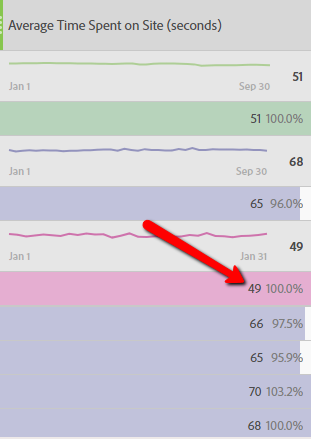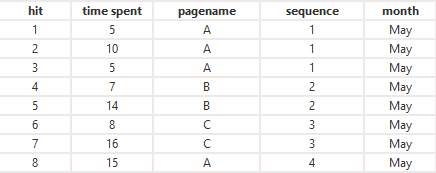## Question

On breaking down dimension with every month for Average Time Spent on Site, the total value is less than the values in each month. Example report:In the above report, the total Average Time Spent on Site is 51 seconds. While the value is never below 60 for each month below. How is the total average value less than 60?

## AnswerConsider the above eight Hits as an example case for this scenario.

Let's calculate Average Time Spent for A. The numerator calculation, that is, time spent is obtained by adding the time spent. The total time spent for A is 5+10+5+15 = 35 seconds. The denominator calculation isn't taking 4 into account but only 2 as it takes sequence in consideration. There were just two sequences (continuous Hit) for A - Hit 1-3 and Hit 8. So, the average time spent for A is 35/2 = 17.5 seconds.

However, the sequence into considered is just 1 on breakdown of dimension with 1. It's because all the Hits are made in the month of May. As a result, the average time spent now broken down by month is 35/1 = 35 seconds which is greater than the average value without breakdown.Twitter™ and Facebook posts are not covered under the terms of Creative Commons.